# Work as a Scalar Product

You may enter values in any of the boxes below. Then click on the symbol for either the scalar product or the angle. The vectors F and d cannot be unambiguously calculated from the scalar product and the angle. If the angle is changed, then d will be placed along the x-axis and F in the xy plane.The scalar product J = ( N)( m)(cos ) degrees.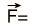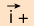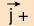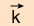Newtons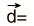meters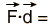()()+()()+()()=Joules
Index

Vector concepts

Work concepts

 HyperPhysics***** Mechanics R Nave
Go Back